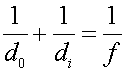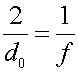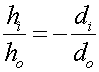Examples for subject

Example #1

Problem:

a.) An image is located at exactly the same position as an object for a mirror of focal length 6 cm. What is the object distance?

Solution:

Use the formulawith di and equal to getdo = 12 cm

b.) If the height of the object is 4.5 mm, what is the height of the image?

Solution:

Useto see that the image height equals the object height.

hi = - 4.5 mm (inverted)

Example #2

Problem:

a.) A convex mirror has an object 14 cm from the mirror, and the image appears to be 7 cm behind the mirror. What is the focal length of the mirror?

Solution:

Use the formulawith the image distance negative.

f = -14 cm

b.) If the object height is 8.0 mm, what is the height of the image?

Solution:

Use the formulawith the image distance negative.

hi = 4.0 cm (upright)

Subject's index